GeeksforGeeks App
Open AppBrowser
Continue

# Increasing and Decreasing Functions

In mathematics, as we know that a function is a relation between input and output. A function can be increasing, decreasing, or constant for the given intervals throughout their entire domain, and they are continuous and differentiable in the given interval. Now, what is an interval: so, an interval is known as a continuous or connected part or portion on the real line. This increasing or decreasing of function is generally used in the application of derivatives. So, if you want to find that the given function is increasing or decreasing in the given interval then you can easily find it with the help of derivatives.

### What is increasing, decreasing, and constant function?

Increasing Function: When a function is increasing in the given interval, then such type of function is known as increasing function. Or in other words, when a function, f(x), is increasing, the values of f(x) are increasing as x increases. Or, let us considered I be an interval which presents in the domain of a real valued function f. Then the function f is increasing on I, if x1 < x2 in I ⇒ f(x1) < f(x2) ∀ x1, x2 ∈ I. Or, in terms of derivative, a function is increasing when the derivative at that point is positive. The graphical representation of an increasing function is: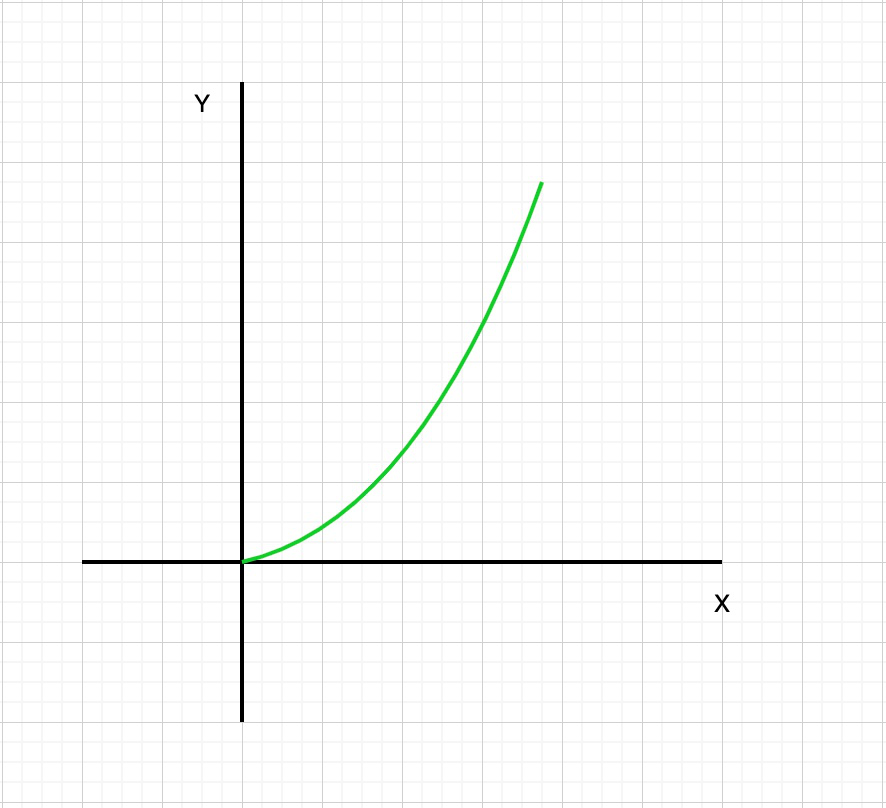Decreasing Function: When a function is decreasing in the given interval, then such type of function is known as decreasing function. Or in other words, when a function, f(x), is decreasing, the values of f(x) are decreasing as x increases. Or, let us considered I be an interval which presents in the domain of a real valued function f. Then

• The function f is decreasing on I, if x1, x2 in I ⇒ f(x1) < f(x2) ∀ x1, x2 ∈ I.
• The function f is decreasing on I, if x1 < x2 in I ⇒ f (x1) ≥ f(x2)∀ x1, x2 ∈ I.
• The function f is strictly decreasing on I, if x1 < x2 in I ⇒ f(x1) > f(x2)∀ x1, x2 ∈ I.

Or, in terms of derivative, a function is decreasing when the derivative at that point is negative. The graphical representation of a decreasing function is: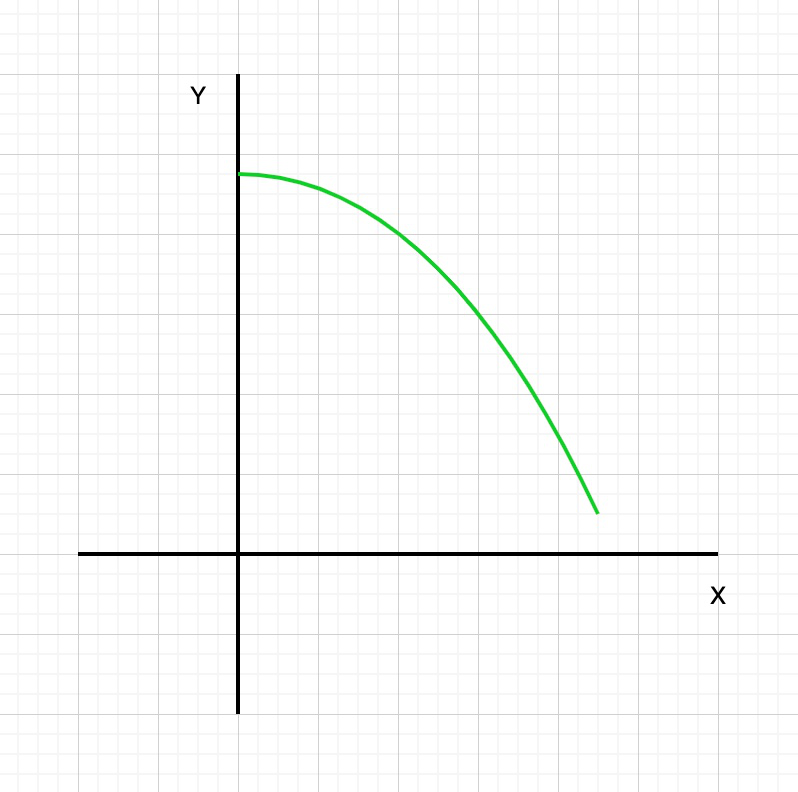Constant function: When a function is neither increasing nor decreasing in the given interval, then such type of function is known as constant function. Or in other words, when a function, f(x), is constant, the value of f(x) does not change as x increases. Or, let us considered I be an interval which presents in the domain of a real valued function f. Then the function f is constant on I, if f(x) = c ∀ x ∈ I. Here, c is a constant. Or, in terms of derivative, a function is constant (i.e. neither increasing nor decreasing) when the derivative is zero. The graphical representation of constant function is: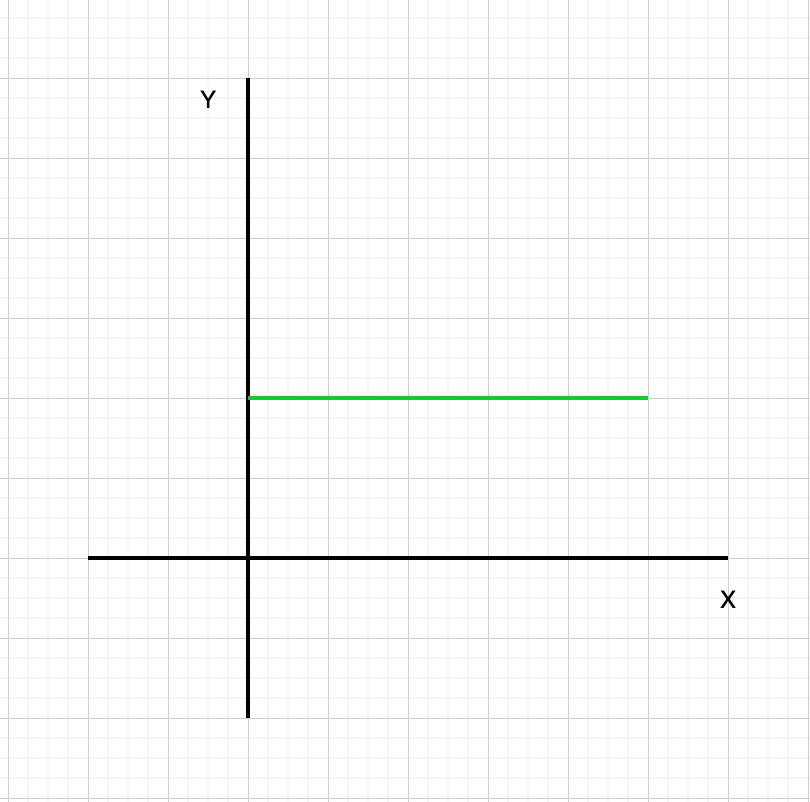Example: In this example, we will investigate the graph of f(x) = x2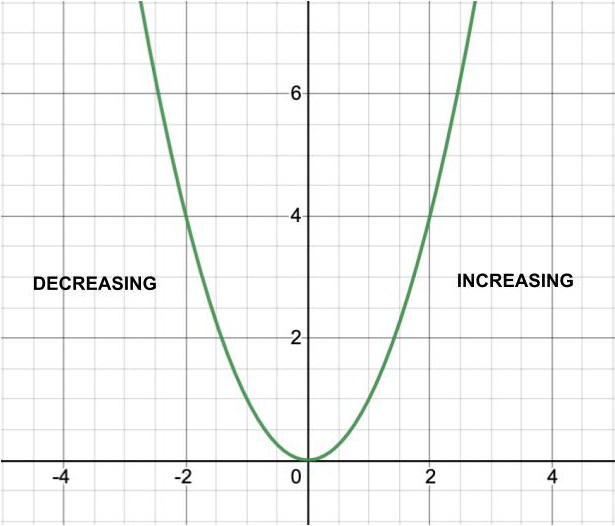Table:

As we can see that when x < 0, the value of f(x) is decreasing as the graph moves to the right. In other words, the “height” of the graph is getting smaller. This is also confirmed by looking at the table of values. When x < 0, as x increases, f(x) decreases. Therefore, f(x) is decreasing on the interval from negative infinity to 0.

When x > 0, the opposite is happening. When x > 0, the value of f(x) is increasing as the graph moves to the right. In other words, the “height” of the graph is getting bigger. This is also confirmed by looking at the table of values. When x > 0, as x increases, f(x) increases. Therefore, f(x) is increasing on the interval from 0 to infinity.

Properties of Increasing & Decreasing Intervals

• Additive property. If the function f and g are increasing/decreasing on the interval (a, b), then the sum of the functions f + g is also increasing/decreasing on this interval.
•  Opposite property. If the function f is increasing/decreasing on the interval (a, b), then the opposite function, -f, is decreasing/increasing.
•  Inverse property. If the function f is increasing/decreasing on the interval (a, b), then the inverse function, 1/f, is decreasing/increasing on this interval.
• Multiplicative property. If the functions f and g are increasing/decreasing and not negative on the interval (a, b), then the product of the functions is also increasing/decreasing.

Theorem:

Let us considered f be a function which is continuous on the interval[p, q] and differentiable on the open interval (p, q), Then

• The function f is increasing in [p, q] if f′(x) > 0 for each x ∈ (p, q).
• The function f is decreasing in [p, q] if f′(x) < 0 for each x ∈ (p, q)
• The function f is constant in [p, q] if f′(x) = 0 for each x ∈ (p, q)

Or

A function y = f(x) is increasing on the interval (p, q) if and only if the first derivative of the function is positive for all x in the interval p < x < q.

Similarly, a function y = f(x) is decreasing on the interval (p, q) if and only if the first derivative of the function is negative for all x in the interval p < x < q.

### Find Increasing and Decreasing Intervals

Given a function, f(x), we can determine the intervals where it is increasing and decreasing by using differentiation and algebra.

Step 1: Find the derivative, f'(x), of the function.

Step 2: Find the zeros of f'(x). Remember, zeros are the values of x for which f'(x) = 0. Set f'(x) = 0 and solve for x.

Step 3: Determine the intervals. The intervals are between the endpoints of the interval of f(x) and the zeros of f'(x). If the interval of f(x) is not given, assume f(x) is on the interval (-∞, ∞).

Step 4: Determine whether the function is increasing or decreasing on each interval. Given the interval (a, c), choose a value b, a < b < c. Solve for f'(b). If f'(b) is positive, f(x) is increasing on (a, c). If f'(b) is negative, f(x) is decreasing on (a, c).

Example 1:

If g(x) = (x – 5)2, find the intervals where g(x) is increasing and decreasing.

Step 1: Find the derivative of the function.

Using the chain rule,

g'(x) = 2(5 – x)

Step 2: Find the zeros of the derivative function.  In other words, find the values of for which g(x) equals zero. You can do this by setting g(x) = 0 and using algebra to solve for x. From the definitions above, we know the function is constant at points where the derivative is zero.

g'(x) = 0 = 2(5 – x)

0 = 5 – x

x = 5

Step 3: Use the zeros to determine intervals.

Since x = 5 is the only zero for g'(x), there are just 2 intervals: from negative infinity to 5, and from 5 to negative infinity.

These can be denoted in inequality notation:

-∞ < x < 5

5 < x < ∞

Or in interval notation:

(-∞, 5)

(5, ∞)

Remember, the endpoints are NOT inclusive because g(x) is neither increasing nor decreasing at the endpoints.

Step 4: Determine if the function is increasing or decreasing in each interval.

For the first interval, ((-∞, 5), we’ll choose b = 0. -∞ < x < 5

g'(b) = g'(0) = 2(5-0) = 10

10 > 0 POSITIVE

For the second interval, (5, ∞), we’ll choose b = 6. 5 < 6 < ∞

g'(b) = g'(6) = 2(5-6) = -2

-2 < 0 NEGATIVE

Therefore, g(x) is increasing on (-∞, 5) and decreasing on (5, ∞). We can verify our results visually. In the graph below, you can clearly see that f(x) = (x – 5)2 is increasing on the interval (5, ∞) and decreasing on the interval (-∞, 5).

We can visually verify our result by investigating the graph of g(x).

Looking at the graph, g(x) is indeed increasing in the interval from negative infinity to 5 and decreasing in the interval from 5 to infinity.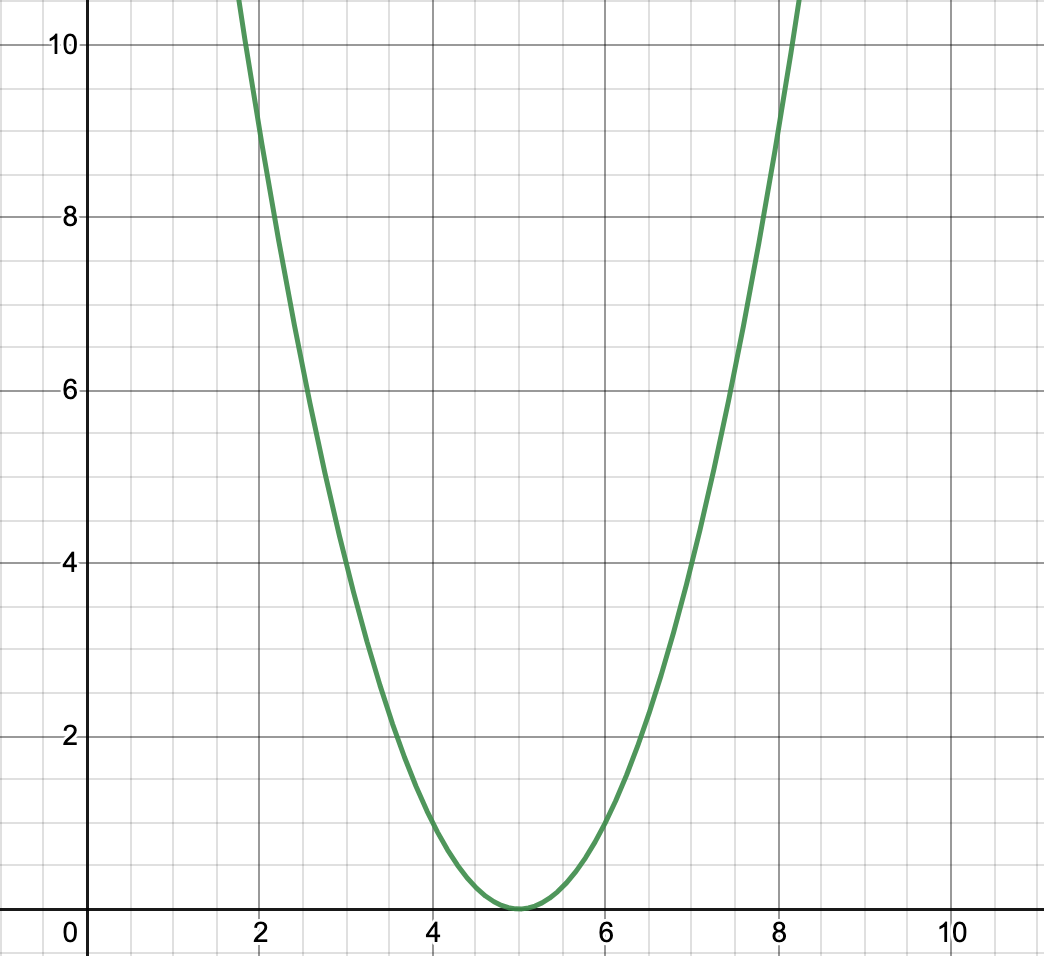Example 2: Find the intervals in -20 < x < 20 where g(x) is increasing and decreasing given g'(x) = x2 – 100.

If the derivative is given, we can skip the first step and go straight to finding the zeroes.

g'(x)= 0 = x2 – 100

x2 = 100Intervals: (-20, -10), (-10, 10), (10, 20)

For (-20, -10), we’ll choose b = -12. -20 < -12 < -10

g'(-12) = 44 > 0

For (-10, 10), we’ll choose b = 0. -10 < 0 < 10

g(0) = -100 < 0

For (10, 20), we’ll choose b = 12. 10 < 12 < 20

g(12) = 44 > 0

Hence, for -20 < x < 20, g(x) is increasing on (-20, -10) and (10, 20) and decreasing on (-10, 10).

We can find g(x) by integrating the given g'(x). Thus,. Looking at the graph, g(x) is indeed increasing in the interval from -20 to -10 and the interval from 10 to 20 and decreasing in the interval from -10 to 10.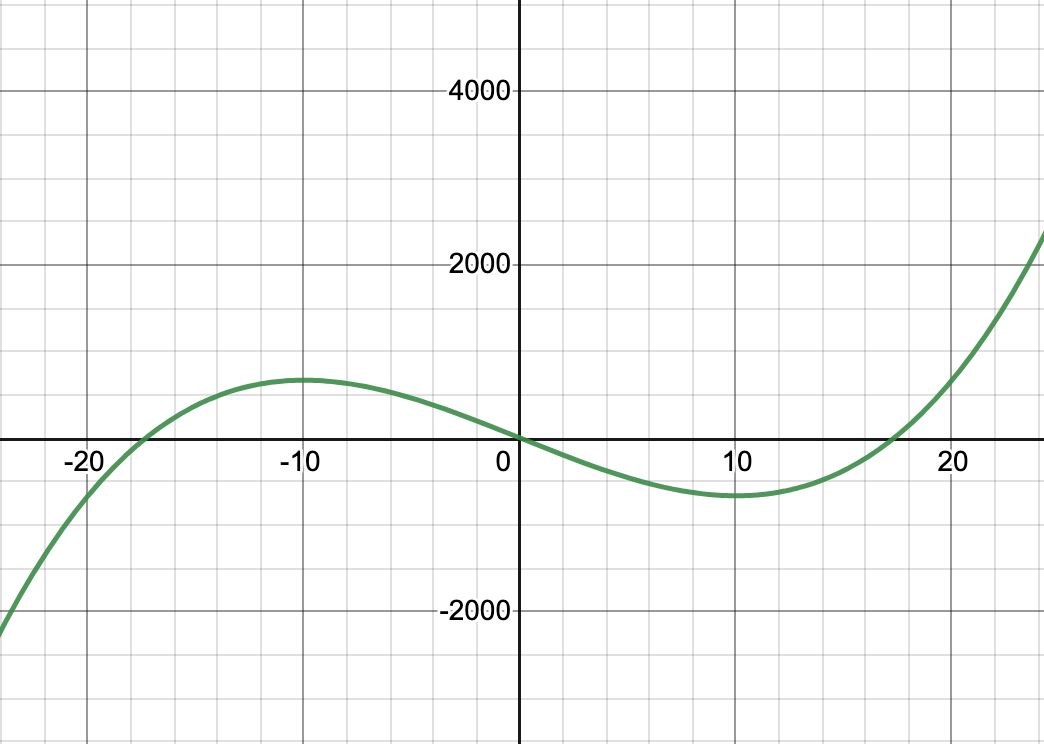### Sample Problems

Question 1. Given the function g(x) = 3x2 – 12, find the intervals on -3 < x < 3 where g(x) is increasing and decreasing.

Solution:

Given function: g(x) = 3x2 – 12

Differentiate w.r.t. x, we get

g'(x) = 6x

For increasing and decreasing

Put g'(x) = 0

g'(x) = 6x = 0

So, x = 0

Intervals: (-3, 0), (0, 3)

At x = -2, g'(-2) = -12 < 0

At x = 2, g'(2) = 12 > 0

So, for -3 < x < 3, g(x) is decreasing on (-3, 0) and increasing on (0, 3).

Question 2. Given the derivative of f(x), f'(x) = -10x2 + 40x, find the intervals where f(x) is increasing and decreasing.

Solution:

Given: f'(x) = -10x2 + 40x

For increasing and decreasing

Put f'(x) = 0

f'(x) =  -10x2 + 40x = 0

So, x = 4, 0

Intervals: (−∞, 0), (0, 4), (4, ∞)

So, at x = -1, f'(-1) = -50 < 0

at x = 1, f'(1) = 30 > 0

at x = 5, f'(5) = -50 < 0

So, f(x) is increasing on (0, 4) and decreasing on (−∞, 0), (4, ∞)

Question 3. Given the function g(x) = 5x2 – 20x + 100, find the intervals where g(x) is increasing and decreasing.

Solution:

Given: g(x) = 5x2 – 20x + 100

Differentiate w.r.t. x, we get

g'(x) = 10x – 20

For increasing and decreasing

Put g'(x) = 0

g'(x) = 10x – 20 = 0

x = 2

Intervals: (−∞, 2), (2, ∞)

At, x = 1, g'(1) = -10 < 0

At x = 3, g'(3) = 10 > 0

So, g(x) is decreasing on (-∞, 2) and increasing on (2, ∞)

Question 4. Given the function s(x) = 6x3 – x2, find the intervals on 0 < x < 10 where s(x) is increasing and decreasing.

Solution:

Given: s(x) = 6x3 – x2

Differentiate w.r.t. x, we get

s'(x) = 18x2 – 2x

For increasing and decreasing

Put s'(x) = 0

s'(x) = 18x2 – 2x = 0

x = 1/9, -1/9

Intervals: (0, 1/9)

Here, -1/9 is not in the given interval, 0 < x < 10

So, g'(1/10) = -0.02 < 0

Hence, for 0 < x < 10, g(x) is decreasing on (0, 1/9)

Question 5. Given g'(x) = 7x2 – 8, find the intervals where g(x) is increasing and decreasing.

Solution:

Given: g'(x) = 7x2 – 8

For increasing and decreasing

Put g'(x) = 0

g'(x) =  7x2 – 8 = 0

x =Intervals:So, At x = -10, g'(-10) = 692 > 0

At x = 0, g'(0) = -8 < 0

At x = 10, g'(10) = 692 < 0

Hence, g(x) is increasing onand, and decreasing onMy Personal Notes arrow_drop_up
Related Tutorials